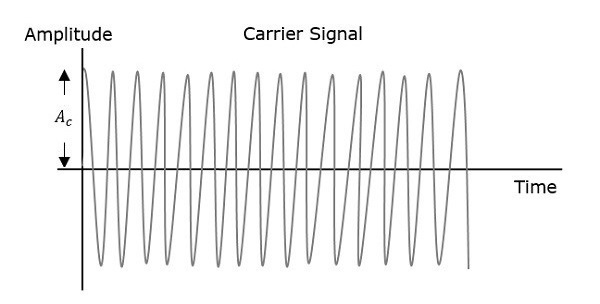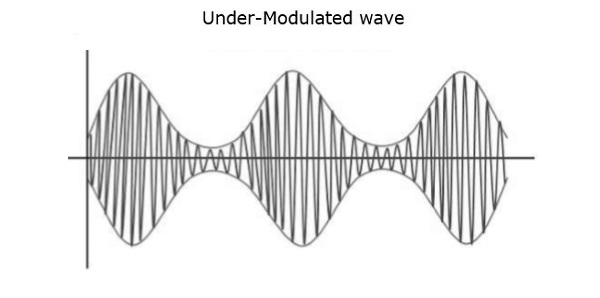# Amplitude Modulation

A continuous-wave goes on continuously without any intervals and it is the baseband message signal, which contains the information. This wave has to be modulated.

According to the standard definition, “The amplitude of the carrier signal varies in accordance with the instantaneous amplitude of the modulating signal.” Which means, the amplitude of the carrier signal containing no information varies as per the amplitude of the signal containing information, at each instant. This can be well explained by the following figures.The first figure shows the modulating wave, which is the message signal. The next one is the carrier wave, which is a high frequency signal and contains no information. While, the last one is the resultant modulated wave.

It can be observed that the positive and negative peaks of the carrier wave, are interconnected with an imaginary line. This line helps recreating the exact shape of the modulating signal. This imaginary line on the carrier wave is called as Envelope. It is the same as that of the message signal.

## Mathematical Expressions

Following are the mathematical expressions for these waves.

### Time-domain Representation of the Waves

Let the modulating signal be,

$$m\left ( t \right )=A_m\cos\left ( 2\pi f_mt \right )$$

and the carrier signal be,

$$c\left ( t \right )=A_c\cos\left ( 2\pi f_ct \right )$$

Where,

$A_m$ and $A_c$ are the amplitude of the modulating signal and the carrier signal respectively.

$f_m$ and $f_c$ are the frequency of the modulating signal and the carrier signal respectively.

Then, the equation of Amplitude Modulated wave will be

$s(t)= \left [ A_c+A_m\cos\left ( 2\pi f_mt \right ) \right ]\cos \left ( 2\pi f_ct \right )$ (Equation 1)

## Modulation Index

A carrier wave, after being modulated, if the modulated level is calculated, then such an attempt is called as Modulation Index or Modulation Depth. It states the level of modulation that a carrier wave undergoes.

Rearrange the Equation 1 as below.

$s(t)=A_c\left [ 1+\left ( \frac{A_m}{A_c} \right )\cos \left ( 2\pi f_mt \right ) \right ]\cos \left ( 2\pi f_ct \right )$

$\Rightarrow s\left ( t \right ) = A_c\left [ 1 + \mu \cos \left ( 2 \pi f_m t \right ) \right ] \cos\left ( 2 \pi f_ct \right )$ (Equation 2)

Where, $\mu$ is Modulation index and it is equal to the ratio of $A_m$ and $A_c$. Mathematically, we can write it as

$\mu = \frac{A_m}{A_c}$ (Equation 3)

Hence, we can calculate the value of modulation index by using the above formula, when the amplitudes of the message and carrier signals are known.

Now, let us derive one more formula for Modulation index by considering Equation 1. We can use this formula for calculating modulation index value, when the maximum and minimum amplitudes of the modulated wave are known.

Let $A_\max$ and $A_\min$ be the maximum and minimum amplitudes of the modulated wave.

We will get the maximum amplitude of the modulated wave, when $\cos \left ( 2\pi f_mt \right )$ is 1.

$\Rightarrow A_\max = A_c + A_m$ (Equation 4)

We will get the minimum amplitude of the modulated wave, when $\cos \left ( 2\pi f_mt \right )$ is -1.

$\Rightarrow A_\min = A_c - A_m$ (Equation 5)

Add Equation 4 and Equation 5.

$$A_\max + A_\min = A_c+A_m+A_c-A_m = 2A_c$$

$\Rightarrow A_c = \frac{A_\max + A_\min}{2}$ (Equation 6)

Subtract Equation 5 from Equation 4.

$$A_\max - A_\min = A_c + A_m - \left (A_c -A_m \right )=2A_m$$

$\Rightarrow A_m = \frac{A_\max - A_\min}{2}$ (Equation 7)

The ratio of Equation 7 and Equation 6 will be as follows.

$$\frac{A_m}{A_c} = \frac{\left ( A_{max} - A_{min}\right )/2}{\left ( A_{max} + A_{min}\right )/2}$$

$\Rightarrow \mu = \frac{A_\max - A_\min}{A_\max + A_\min}$ (Equation 8)

Therefore, Equation 3 and Equation 8 are the two formulas for Modulation index. The modulation index or modulation depth is often denoted in percentage called as Percentage of Modulation. We will get the percentage of modulation, just by multiplying the modulation index value with 100.

For a perfect modulation, the value of modulation index should be 1, which implies the percentage of modulation should be 100%.

For instance, if this value is less than 1, i.e., the modulation index is 0.5, then the modulated output would look like the following figure. It is called as Under-modulation. Such a wave is called as an under-modulated wave.If the value of the modulation index is greater than 1, i.e., 1.5 or so, then the wave will be an over-modulated wave. It would look like the following figure.As the value of the modulation index increases, the carrier experiences a 180o phase reversal, which causes additional sidebands and hence, the wave gets distorted. Such an over-modulated wave causes interference, which cannot be eliminated.

## Bandwidth of AM Wave

Bandwidth (BW) is the difference between the highest and lowest frequencies of the signal. Mathematically, we can write it as

$$BW = f_{max} - f_{min}$$

Consider the following equation of amplitude modulated wave.

$$s\left ( t \right ) = A_c\left [ 1 + \mu \cos \left ( 2 \pi f_m t \right ) \right ] \cos\left ( 2 \pi f_ct \right )$$

$$\Rightarrow s\left ( t \right ) = A_c\cos \left ( 2\pi f_ct \right )+ A_c\mu \cos(2\pi f_ct)\cos \left ( 2\pi f_mt \right )$$

$\Rightarrow s\left ( t \right )= A_c\cos \left ( 2\pi f_ct \right )+\frac{A_c\mu }{2}\cos \left [ 2\pi \left ( f_c+f_m \right ) t\right ]+\frac{A_c\mu }{2}\cos \left [ 2\pi \left ( f_c-f_m \right ) t\right ]$

Hence, the amplitude modulated wave has three frequencies. Those are carrier frequency $f_c$, upper sideband frequency $f_c + f_m$ and lower sideband frequency $f_c-f_m$

Here,

$f_{max}=f_c+f_m$ and $f_{min}=f_c-f_m$

Substitute, $f_{max}$ and $f_{min}$ values in bandwidth formula.

$$BW=f_c+f_m-\left ( f_c-f_m \right )$$

$$\Rightarrow BW=2f_m$$

Thus, it can be said that the bandwidth required for amplitude modulated wave is twice the frequency of the modulating signal.

## Power Calculations of AM Wave

Consider the following equation of amplitude modulated wave.

$\ s\left ( t \right )= A_c\cos \left ( 2\pi f_ct \right )+\frac{A_c\mu }{2}\cos \left [ 2\pi \left ( f_c+f_m \right ) t\right ]+\frac{A_c\mu }{2}\cos \left [ 2\pi \left ( f_c-f_m \right ) t\right ]$

Power of AM wave is equal to the sum of powers of carrier, upper sideband, and lower sideband frequency components.

$$P_t=P_c+P_{USB}+P_{LSB}$$

We know that the standard formula for power of cos signal is

$$P=\frac{{v_{rms}}^{2}}{R}=\frac{\left ( v_m/ \sqrt{2}\right )^2}{2}$$

Where,

$v_{rms}$ is the rms value of cos signal.

$v_m$ is the peak value of cos signal.

First, let us find the powers of the carrier, the upper and lower sideband one by one.

Carrier power

$$P_c=\frac{\left ( A_c/\sqrt{2} \right )^2}{R}=\frac{{A_{c}}^{2}}{2R}$$

Upper sideband power

$$P_{USB}=\frac{\left ( A_c\mu /2\sqrt{2} \right )^2}{R}=\frac{{A_{c}}^{2}{_{\mu }}^{2}}{8R}$$

Similarly, we will get the lower sideband power same as that of the upper side band power.

$$P_{LSB}=\frac{{A_{c}}^{2}{_{\mu }}^{2}}{8R}$$

Now, let us add these three powers in order to get the power of AM wave.

$$P_t=\frac{{A_{c}}^{2}}{2R}+\frac{{A_{c}}^{2}{_{\mu }}^{2}}{8R}+\frac{{A_{c}}^{2}{_{\mu }}^{2}}{8R}$$

$$\Rightarrow P_t=\left ( \frac{{A_{c}}^{2}}{2R} \right )\left ( 1+\frac{\mu ^2}{4}+\frac{\mu ^2}{4} \right )$$

$$\Rightarrow P_t=P_c\left ( 1+\frac{\mu ^2}{2} \right )$$

We can use the above formula to calculate the power of AM wave, when the carrier power and the modulation index are known.

If the modulation index $\mu=1$ then the power of AM wave is equal to 1.5 times the carrier power. So, the power required for transmitting an AM wave is 1.5 times the carrier power for a perfect modulation.

## Useful Video Courses

Video

#### Read Analog Sensors with Arduino

13 Lectures 1 hours